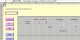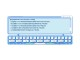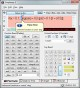Spreadsheet Composer 2.0 An Excel add-in for automatic construction and management of complex formula. FreewareMy Talking Calculator 4.18 A talking calculation robot,has the huge float number calculator. SharewareSimplexety 8.0.0.1 Simplexety, Editor and VBScript Engine. FreewareQuadratic Formula Helper 0.1 Quadratic Formula Helper is a small application for quickly finding the value(s) of X from a quadratic formula. FreewareAspose.Cells for .NET 8.1.1.0 Aspose.Cells is a .NET component for spreadsheet reporting. SharewareDynamic Formula 1.0 Dynamic Formula is a RbScript subclass which can be used to allow end users to enter in their own variables and values which you, the developer, can use as a dynamic formula which is embedded into your code. FreewareQuadratic Formula 1.1 Quadratic Formula is a simple program that will solve the Quadratic Formula for you. FreewareCalcPad 1.0.1 Type the formula in the window, then push [enter] or [return]. FreewareFractographer 2.0.3 Fractographer is a program to plot the fractals of mathematical functions. FreewareStupor 1.0 Stupor is based on a simple formula. Freeware